# Preliminary steps

Start by loading the necessary packages (which must be installed beforehand if they are not already installed) : TraMineR and TraMineRextras for sequence analysis, cluster and WeightedCluster for clustering, FactoMineR et ade4 for correspondence analysis, RColorBrewer for color palettes, questionr et descriptio for descriptive statistics, dplyr et purrr for data manipulation, ggplot2 for plots and seqhandbook which accompanies the handbook.

library(TraMineR)
library(TraMineRextras)
library(cluster)
library(WeightedCluster)
library(FactoMineR)
library(RColorBrewer)
library(questionr)
library(descriptio)
library(dplyr)
library(purrr)
library(ggplot2)
library(seqhandbook)

The data used in the handbook is then loaded. The data on employment trajectories are in the data frame trajact: there are 500 observations, i.e. 500 individual trajectories, and 37 variables, corresponding to the activity status observed each year between the ages of 14 and 50.

# loading the trajectories
data(trajact)
str(trajact)
'data.frame':   500 obs. of  37 variables:
$sact14: num 2 1 1 1 1 1 1 1 1 1 ...$ sact15: num  2 1 1 1 1 1 1 1 1 1 ...
$sact16: num 2 1 1 1 1 1 1 1 1 1 ...$ sact17: num  2 1 1 1 1 1 2 2 2 2 ...
$sact18: num 2 1 2 2 1 1 2 2 2 2 ...$ sact19: num  2 3 2 2 1 1 2 2 2 2 ...
$sact20: num 2 3 2 6 2 2 5 2 6 2 ...$ sact21: num  2 3 2 2 2 2 5 6 6 2 ...
$sact22: num 2 3 2 2 2 2 5 6 2 2 ...$ sact23: num  2 3 2 2 2 2 5 2 2 2 ...
$sact24: num 2 3 2 2 2 5 3 2 2 2 ...$ sact25: num  2 3 2 2 2 5 3 2 2 2 ...
$sact26: num 2 3 2 2 2 5 3 2 2 2 ...$ sact27: num  2 3 2 2 2 2 3 2 2 2 ...
$sact28: num 2 3 5 2 2 2 3 2 2 2 ...$ sact29: num  2 3 2 2 2 2 3 2 2 2 ...
$sact30: num 2 3 5 2 2 2 3 2 2 2 ...$ sact31: num  2 3 5 2 2 2 3 2 2 2 ...
$sact32: num 2 3 5 2 2 2 5 2 2 2 ...$ sact33: num  2 3 5 2 2 2 5 2 2 2 ...
$sact34: num 2 3 5 2 2 2 5 2 2 2 ...$ sact35: num  2 3 5 2 2 2 2 2 2 2 ...
$sact36: num 2 3 5 2 2 2 2 2 2 2 ...$ sact37: num  2 3 5 2 2 2 2 2 2 2 ...
$sact38: num 2 2 5 2 2 2 2 2 2 2 ...$ sact39: num  2 2 5 2 2 2 2 2 2 2 ...
$sact40: num 2 2 5 2 2 2 2 2 2 2 ...$ sact41: num  2 2 5 2 2 2 2 2 2 2 ...
$sact42: num 2 2 5 2 2 2 2 2 2 2 ...$ sact43: num  2 2 5 2 2 2 2 2 2 2 ...
$sact44: num 2 2 5 2 2 2 2 2 2 2 ...$ sact45: num  2 2 3 2 2 2 2 2 2 2 ...
$sact46: num 2 2 3 2 2 2 2 2 2 2 ...$ sact47: num  2 2 3 2 2 2 2 2 2 2 ...
$sact48: num 2 2 3 2 2 2 2 2 2 2 ...$ sact49: num  2 2 3 2 2 2 2 2 2 2 ...
$sact50: num 2 3 3 2 2 2 2 2 2 2 ... The first step in sequence analysis is to create a corpus of sequences, i.e. a stslist class object using the seqdef function. First the labels of the 6 states and a palette of 6 colours are defined (this is optional: seqdef creates labels and palette automatically if not provided). # defining of a corpus of sequences labs <- c("studies","full-time","part-time","short jobs","inactivity","military") palette <- brewer.pal(length(labs), 'Set2') seqact <- seqdef(trajact, labels=labs, cpal=palette) Our corpus of 500 sequences consists of 377 distinct sequences, which confirms the interest of using a statistical procedure to group together sequences that are similar. # number of distinct sequences seqtab(seqact, idx=0) %>% nrow  377 The state distribution plot of all the sequences shows the preponderance of full-time employment and the non-negligible weight of inactivity. # state distribution plot seqdplot(seqact, xtlab=14:50, cex.legend=0.7)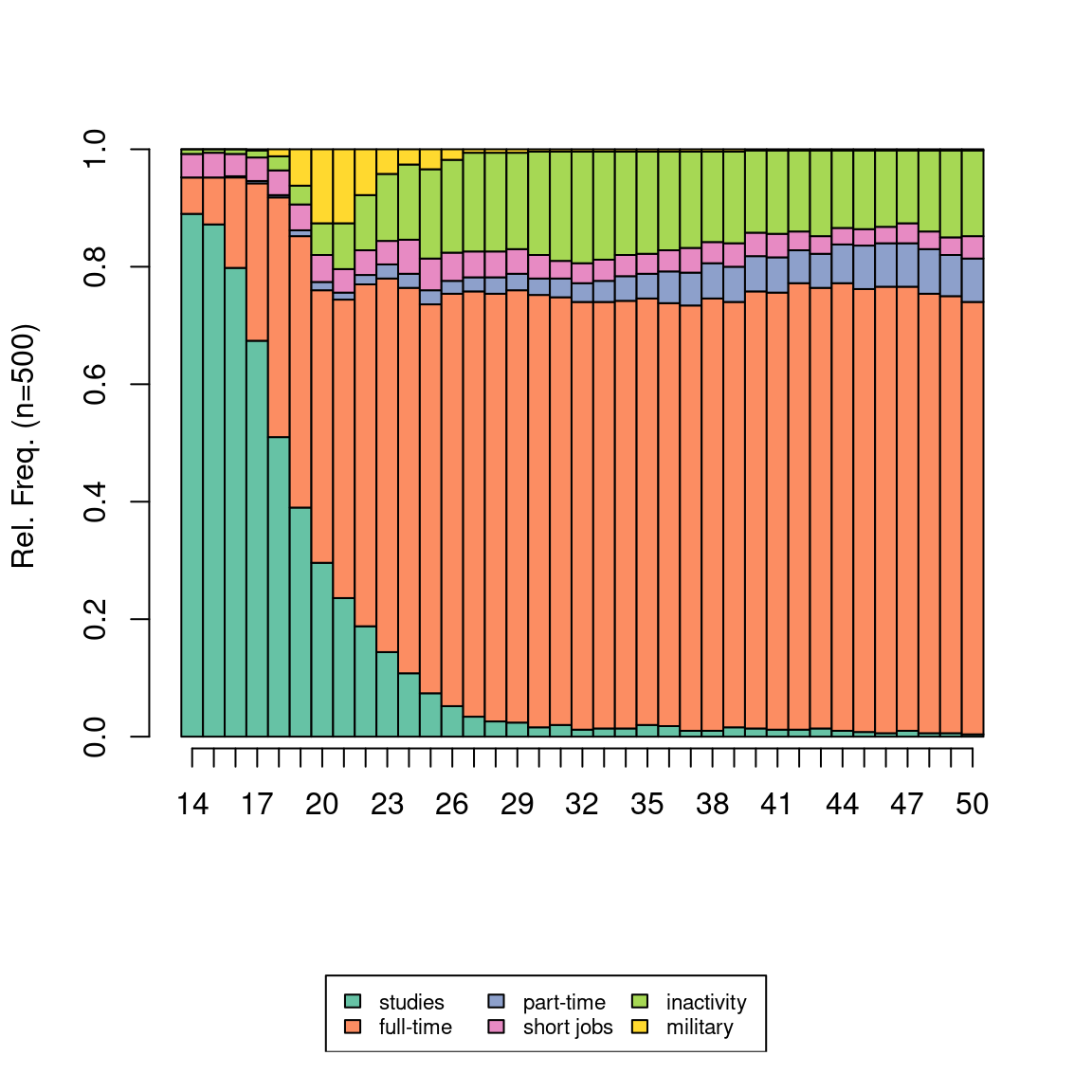A data frame is also loaded containing some socio-demographic variables on the individuals. Note that the categorical variables are in factor format. # loading the socio-demographic variables data(socdem) str(socdem) 'data.frame': 500 obs. of 9 variables:$ annais     : num  1950 1937 1933 1948 1944 ...
$nbenf : Factor w/ 4 levels "aucun","un","deux",..: 4 3 3 3 3 2 4 4 4 1 ...$ nbunion    : Factor w/ 3 levels "aucune","une",..: 2 2 2 2 3 2 2 2 2 1 ...
$mereactive : Factor w/ 2 levels "non","oui": 1 1 2 2 1 1 2 1 1 2 ...$ sexe       : Factor w/ 2 levels "homme","femme": 1 2 2 1 2 2 2 1 1 2 ...
$PCS : Factor w/ 8 levels "NA","agric","indep",..: 7 8 5 7 6 5 6 7 7 4 ...$ PCSpere    : Factor w/ 8 levels "agric","indep",..: 7 1 5 2 6 3 6 5 6 6 ...
$diplome : Factor w/ 4 levels "aucun","<bac",..: 1 2 2 2 3 4 2 2 2 2 ...$ nationalite: Factor w/ 2 levels "francaise","etrangere": 1 1 1 1 1 1 1 1 1 1 ...

# Building a distance matrix

## Synthetic indicators

As an example, a distance matrix is constructed from indicators describing the number of episodes in the different states. The first individual has spent his entire trajectory in full-time employment, while the second has experienced one full-time episode but also one episode of study and two of part-time employment.

indics <- seqinepi(seqact)
head(indics)
  nepi1 nepi2 nepi3 nepi4 nepi5 nepi6
1     0     1     0     0     0     0
2     1     1     2     0     0     0
3     1     2     1     0     2     0
4     1     2     0     0     0     1
5     1     1     0     0     0     0
6     1     2     0     0     1     0

The matrix can be calculated directly from the indicators or after a Principal Component Analysis (PCA) step, here by retaining the first 5 dimensions.

# distance matrix from the indicator
dissim <- dist(indics, method='euclidean') %>% as.matrix

# distance matrix from PCA results
acp_coords <- PCA(indics, scale.unit=FALSE, ncp=5, graph=FALSE)$ind$coord
dissim <- dist(acp_coords, method='euclidean') %>% as.matrix

Other synthetic indicators (durations, states visited, etc.) can be calculated simply from the functions seqistatd, seqi1epi, seqifpos, seqindic or seqpropclust.

## Disjunctive coding and QHA

In the case of complete disjunctive coding, the distance matrix can be calculated directly, with the Euclidean distance or the chi2 distance, or after a Principal Component Analysis (PCA) or Multiple Correspondence Analysis (MCA) step, here by retaining the first 5 dimensions.

NB: map_df allows you to apply the same function to all the columns of a data frame. Here, this function is used to convert columns from numerical format to factor format.

# complete disjunctive coding
disjo <- as.data.frame(tab.disjonctif(seqact))
disjo <- disjo[,colSums(disjo)>0]

# euclidian distance
dissim <- dist(disjo, method='euclidean') %>% as.matrix

# chi2 distance
dissim <- map_df(disjo, as.factor) %>%
dudi.acm(scannf=FALSE, nf=ncol(disjo)) %>%
dist.dudi() %>%
as.matrix

# after a PCA step
acp_coords <- PCA(disjo, scale.unit=FALSE, ncp=5, graph=FALSE)$ind$coord
dissim <- dist(acp_coords, method='euclidean') %>% as.matrix

# after a MCA step
acm_res <- purrr::map_df(disjo, as.factor) %>%
MCA(ncp=5, graph=FALSE)
dissim <- dist(acm_res$ind$coord, method='euclidean') %>% as.matrix

For the qualitative harmonic analysis (QHA), the calculation of the distance matrix can be done directly (chi2 distance) or after a correspondence analysis (CA), here using the first 5 dimensions.

# QHA coding
ahq <- seq2qha(seqact, c(1,3,7,10,15,20,28))
ahq <- ahq[,colSums(ahq)>0]

# chi2 distance
dissim <- dudi.coa(ahq, scannf=FALSE, nf=ncol(ahq)) %>%
dist.dudi() %>%
as.matrix

# after a CA step
afc_coord <- CA(ahq, ncp=5, graph=FALSE)$row$coord
dissim <- dist(afc_coord, method='euclidean') %>% as.matrix

## Optimal Matching and alternatives

For Optimal Matching, the construction of a distance matrix between sequences is done with the seqdist function. This also involves defining a substitution cost matrix between states (with the seqsubm function). Here the substitution costs are constant and equal to 2 and the indel cost is equal to 1.5.

# building the distance matrix
couts <- seqsubm(seqact, method="CONSTANT", cval=2)
dissim <- seqdist(seqact, method="OM", sm=couts, indel=1.5)

From experience, Optimal Matching with the parameters adopted here is a choice that allows the different dimensions of sequence temporality to be taken into account - sequencing, calendar (timing), duration (in different states or episodes, duration and spell duration). If you wish to focus on one of these dimensions, you can follow the recommendations of Studer & Ritschard (2016, see in particular pages 507-509), and choose one of the many other metrics implemented in the TraMineR extension.

# sequencing
dissim <- seqdist(seqact, method="OMstran", otto=0.1, sm=couts, indel=1)
dissim <- seqdist(seqact, method="OMspell", expcost=0, sm=couts, indel=1)
dissim <- seqdist(seqact, method="SVRspell", tpow=0)

# timing
dissim <- seqdist(seqact, method="HAM", sm=couts)
dissim <- seqdist(seqact, method="CHI2", step=1)

# duration (distribution aver the entire period)
dissim <- seqdist(seqact, method="EUCLID", step=37)

# duration (spell lengths)
dissim <- seqdist(seqact, method="OMspell", expcost=1, sm=couts, indel=1)
dissim <- seqdist(seqact, method="LCS")

Note that methods using the complete disjunctive coding or QHA are also implemented in the seqdist function (“EUCLID” and “CHI2” methods).

# Typology of sequences

## Building a typology

A hierarchical agglomerative clustering (HAC) is then carried out using Ward’s aggregation criterion, using the agnes function of the cluster extension.

NB: With a high number of sequences, HAC may require a significant amount of computing time. However, there is a much faster implementation in the fastcluster package (hclust function).

# hierarchical agglomerative clustering
agnes <- as.dist(dissim) %>% agnes(method="ward", keep.diss=FALSE)

In order to explore the solutions of a hierarchical agglomerative clustering, one usually starts by examining the dendrogram.

# dendrogram
as.dendrogram(agnes) %>% plot(leaflab="none")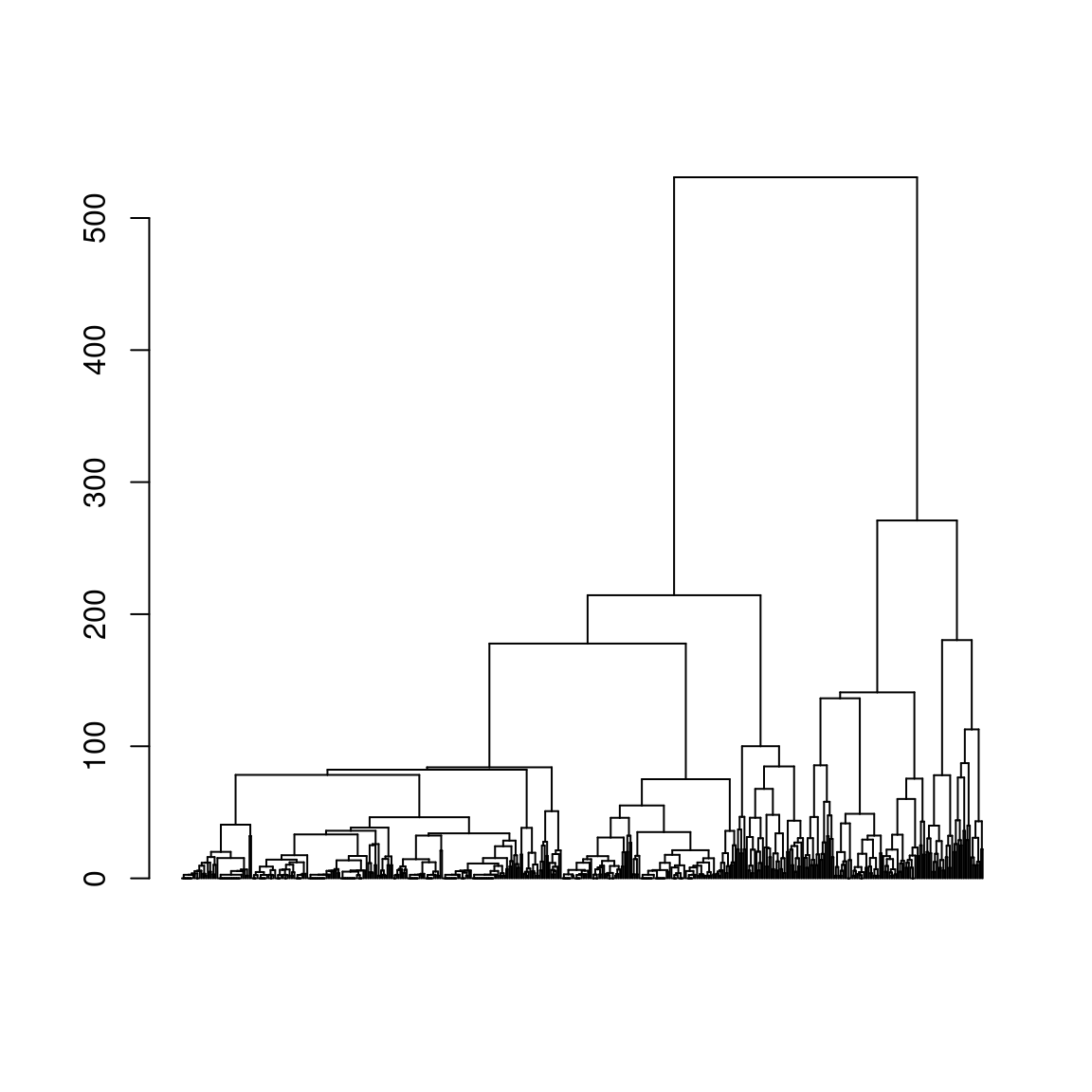The following graph combines dendrogram and index plot: the sequences of the index plot index are sorted according to their position in the dendrogram, which is shown in the margin of the graph.

# heatmap (dendrogram + index plot)
seq_heatmap(seqact, agnes)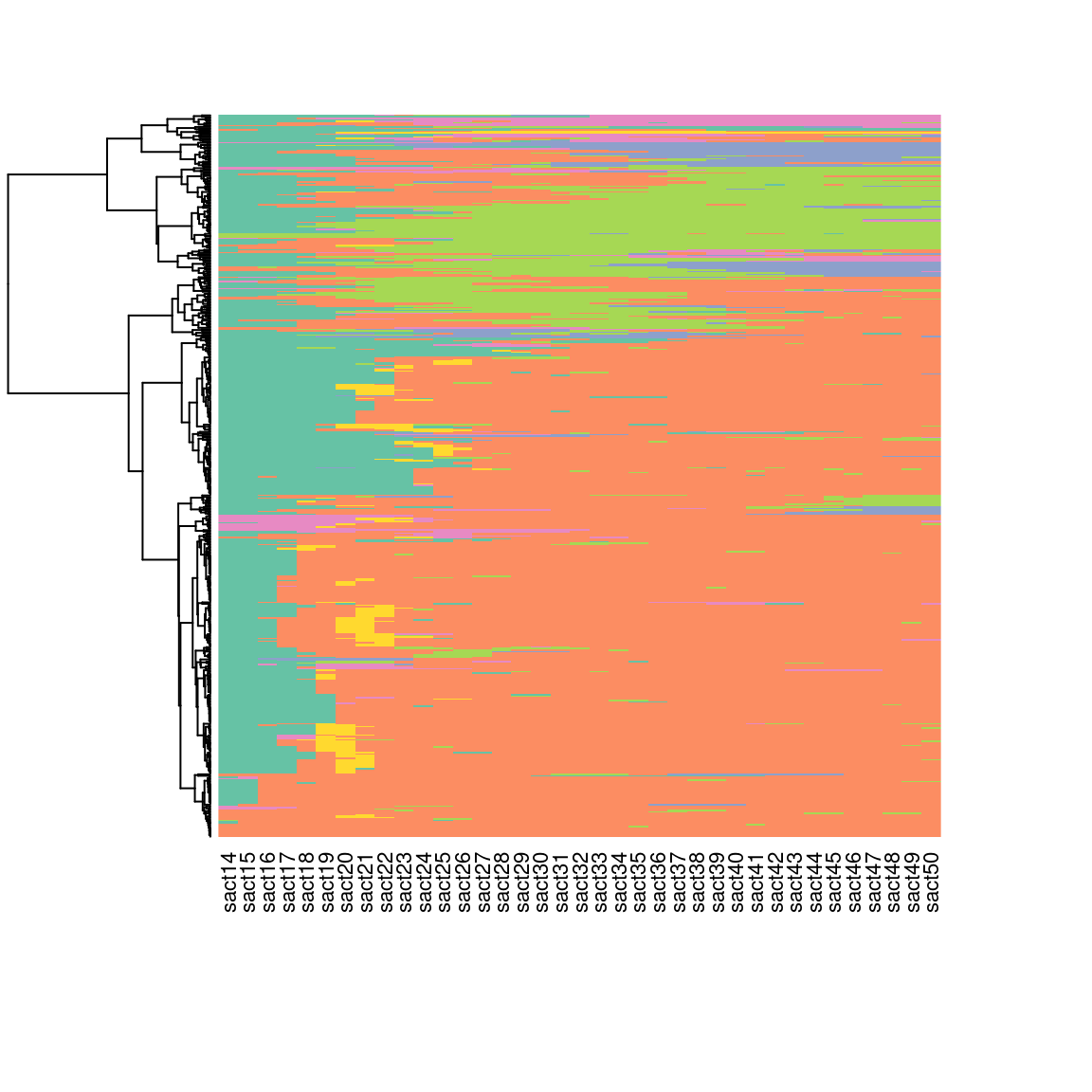Examination of inertia gaps can also be useful in determining the number of clusters in the typology. For example, it can be seen that there is a noticeable difference in inertia between partitions in 5 and 6 clusters.

# plot of inertia
plot(sort(agnes$height, decreasing=TRUE)[1:20], type="s", xlab="number of clusters", ylab="inertia")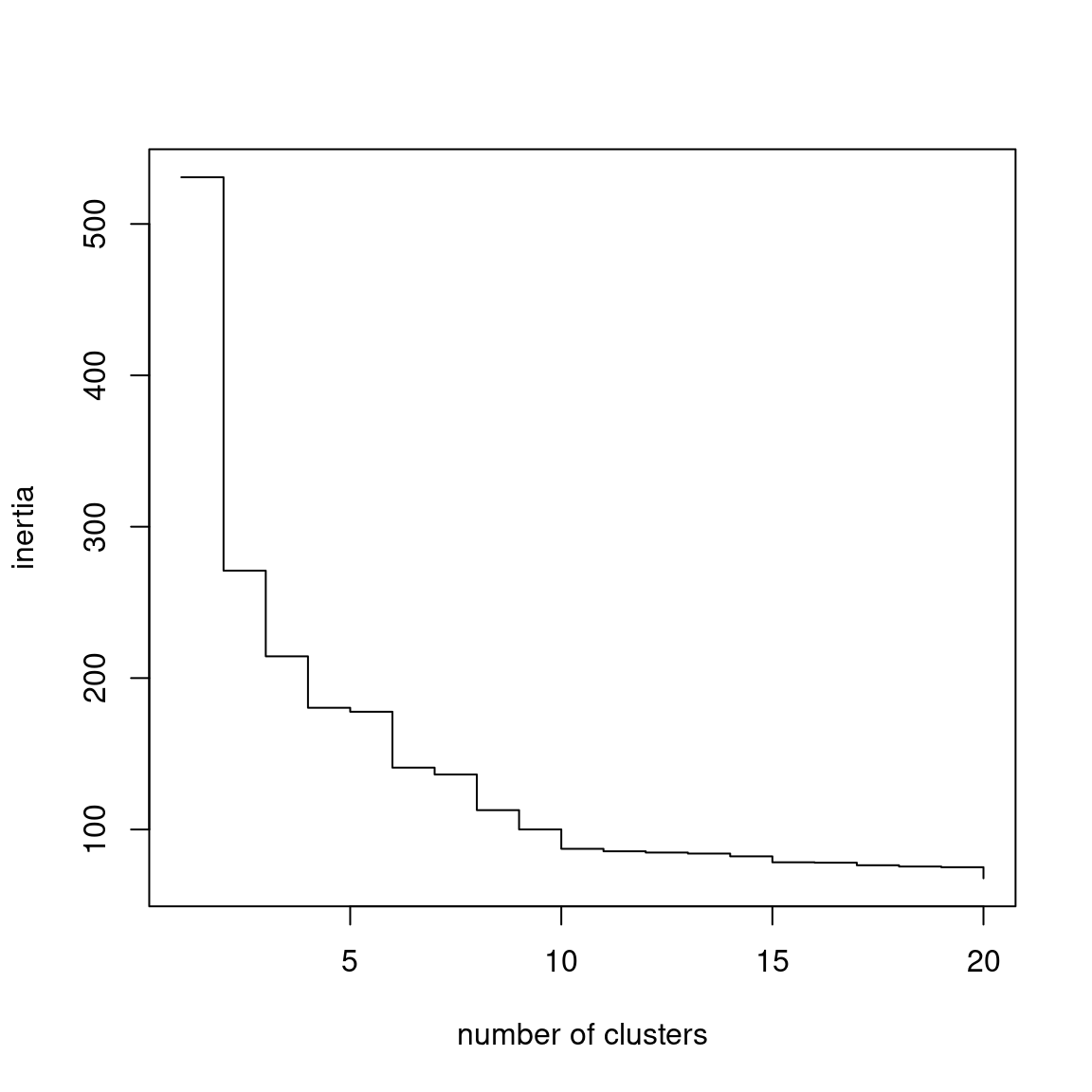There are also a number of indicators of partition quality (silhouette, Calinski-Harabasz, pseudo-R2, etc.; see Studer, 2013). # indicators of quality wardRange <- as.clustrange(agnes, diss=dissim) summary(wardRange, max.rank=2)  1. N groups 1. stat 2. N groups 2. stat PBC 5 0.79612318 4 0.79012613 HG 5 0.92899997 4 0.92259604 HGSD 5 0.92672338 4 0.92027518 ASW 3 0.53491169 2 0.52958820 ASWw 3 0.53862662 2 0.53182999 CH 2 156.86409716 3 103.74539004 R2 20 0.60648740 19 0.59473652 CHsq 2 324.53910100 3 245.89231855 R2sq 20 0.82862437 19 0.82073439 HC 5 0.04010082 4 0.04392402 The quality of the partitions for different numbers of clusters for the silhouette, pseudo-R2 and Calinski-Harabasz indicators is shown graphically here. plot(wardRange, stat=c('ASW','R2','CH'), norm="zscore")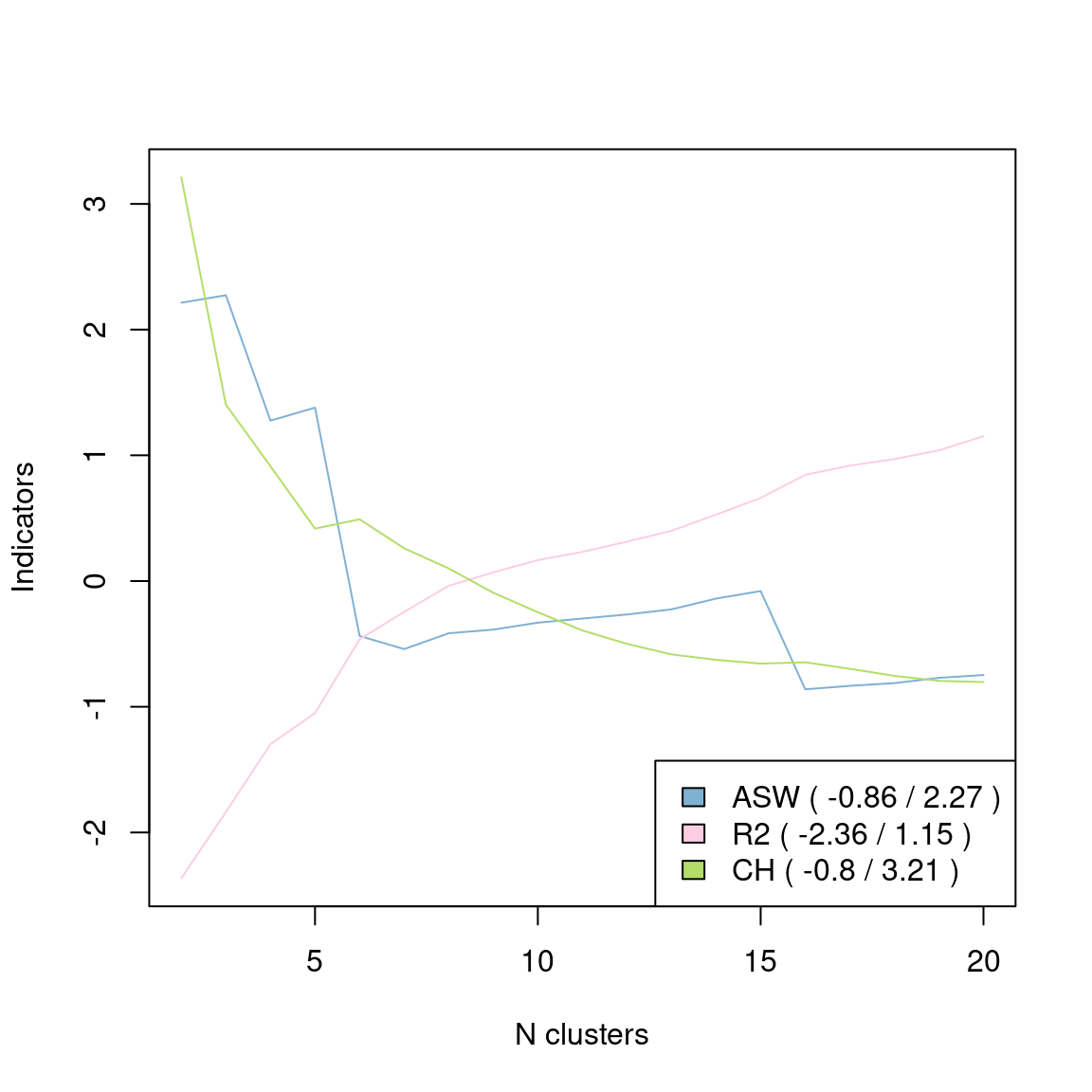In the end, we opt for a partition in 5 clusters, by “cutting the tree” of the HAC using the cutree function. # choosing the partition in 5 clusters nbcl <- 5 part <- cutree(agnes, nbcl) It is possible to “consolidate” the partition using the PAM algorithm (Partition Around Medoids) and the wcKMedoids function of the WeightedCluster package. This results in a very similar distribution of sequences between the clusters (see the table crossing the clusters before and after consolidation) but the quality of the consolidated partition is slightly higher (the R² goes from 61 to 64%). # consolidating the partition newpart <- wcKMedoids(dissim, k=nbcl, initialclust=part, cluster.only=TRUE) table(part, newpart)  newpart part 28 34 103 307 480 1 104 241 1 0 0 2 9 24 2 7 0 3 0 6 6 63 1 4 0 0 17 0 0 5 8 0 0 1 10 wcClusterQuality(dissim, part)$stats['R2sq'] %>% round(3)
 R2sq
0.607 
wcClusterQuality(dissim, newpart)$stats['R2sq'] %>% round(3) R2sq 0.64  If you wish to keep the consolidated partition : part <- as.numeric(as.factor(newpart)) NB: Another option, the “fuzzy” clustering, here with the Fanny algorithm (extension cluster). Unlike HAC or PAM, each sequence does not belong to a single cluster but is characterised by degrees of membership to the different clusters. The following table presents the degrees of membership to the 6 clusters of the first 3 sequences of the corpus. The first sequence belongs 99% to cluster 1, but the second is more “balanced”, mainly between clusters 2 and 5. # fuzzy clustering fanny <- as.dist(dissim) %>% fanny(k=5, metric='euclidean', memb.exp=1.2) fanny$membership %>% round(2) %>% .[1:3,]
  [,1] [,2] [,3] [,4] [,5]
1 0.99 0.00 0.00 0.01 0.00
2 0.06 0.51 0.02 0.13 0.28
3 0.00 0.11 0.80 0.00 0.08

## Describing the typology: plots

The graphical representations give a quick and intuitive idea of the nature of the clusters in the typology. The most commonly used type of graph is the state distribution plot.

# state distribution plots of the typology
seqdplot(seqact, group=part, xtlab=14:50, border=NA, cex.legend=0.8)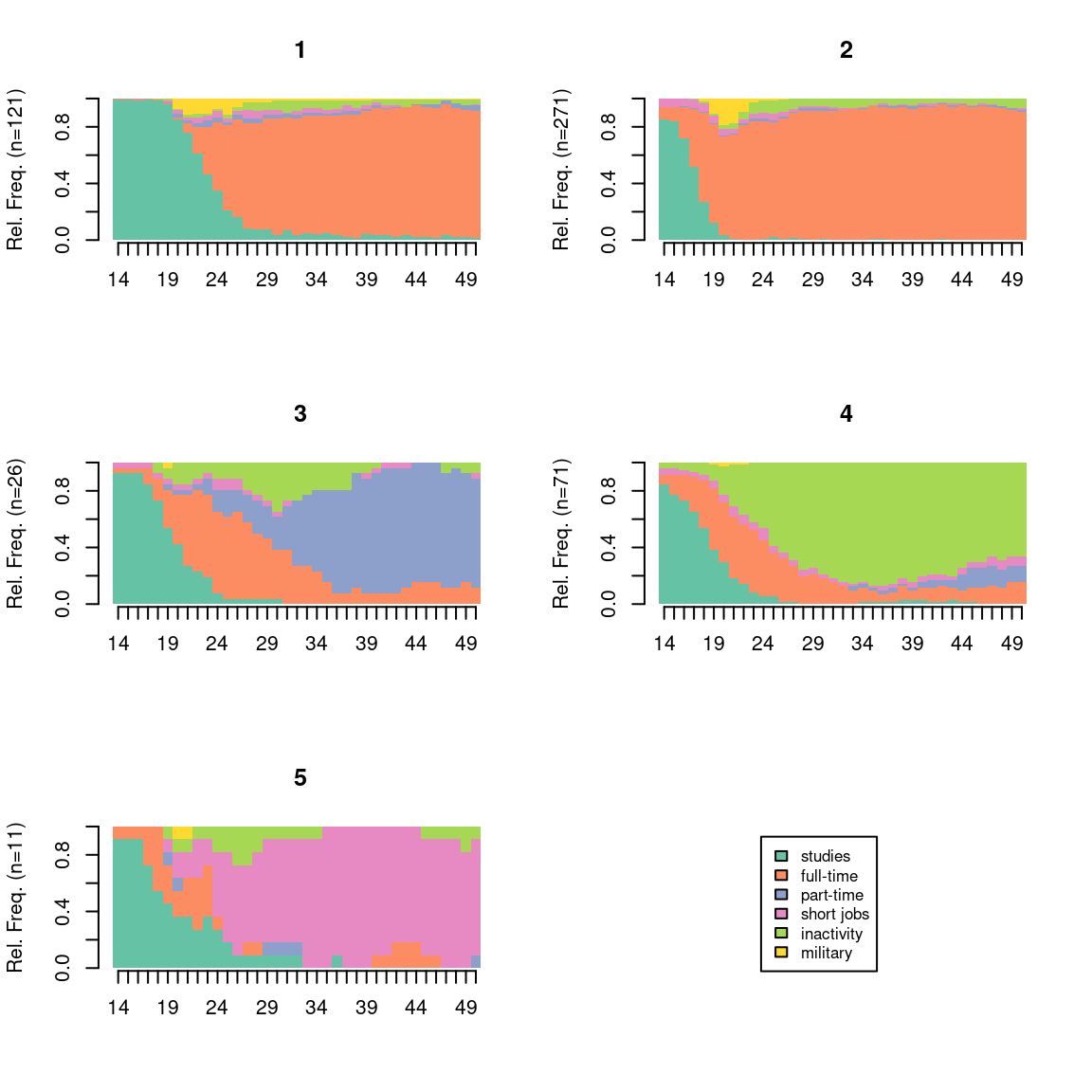The index plots are also very common.

# index plots of the typology
seqIplot(seqact, group=part, xtlab=14:50, yaxis=FALSE, cex.legend=0.8)